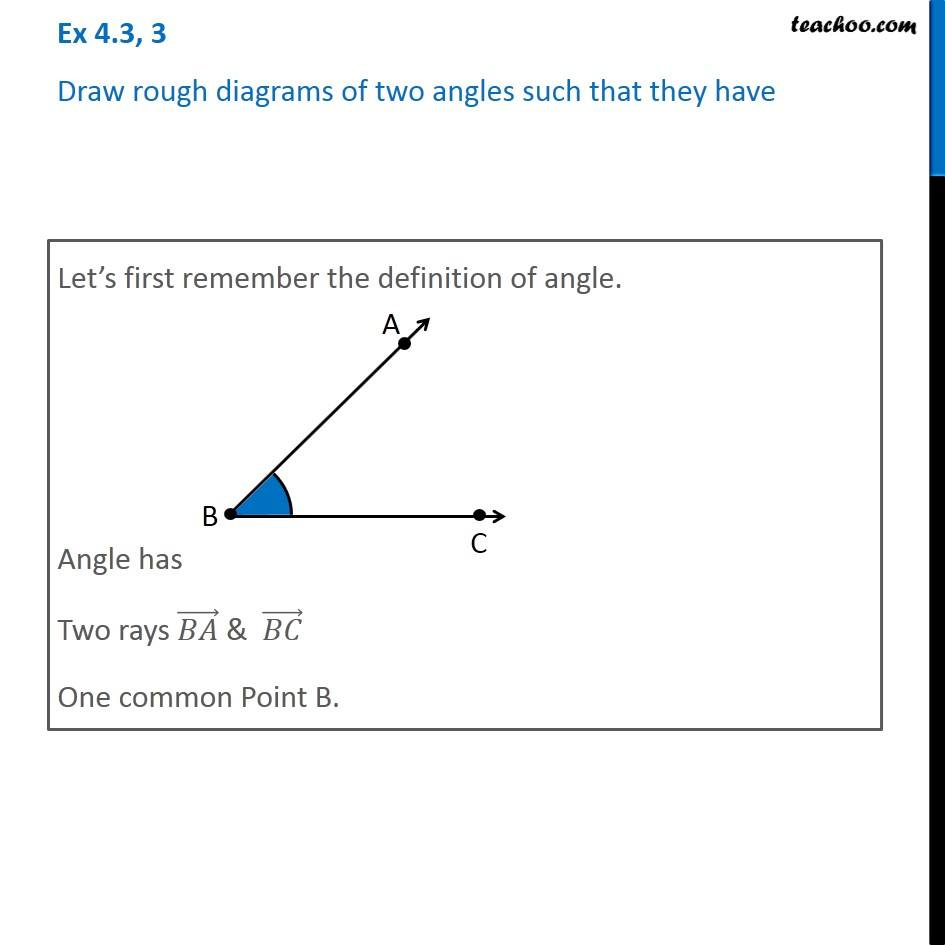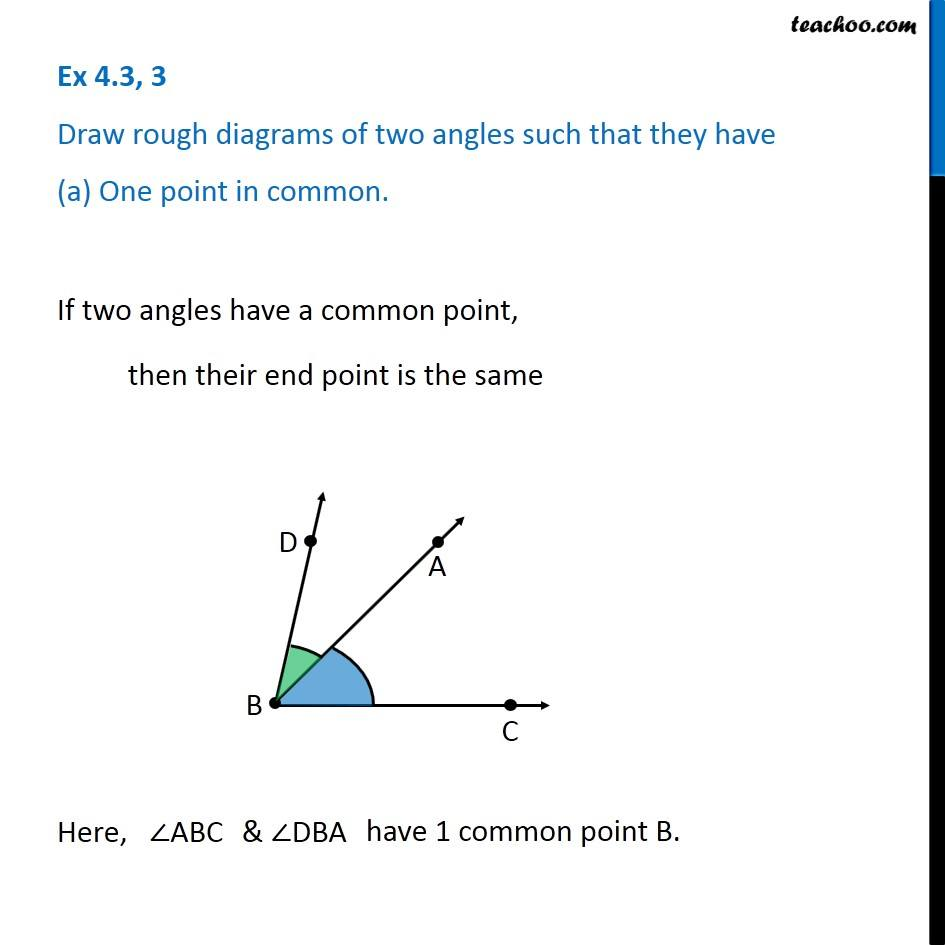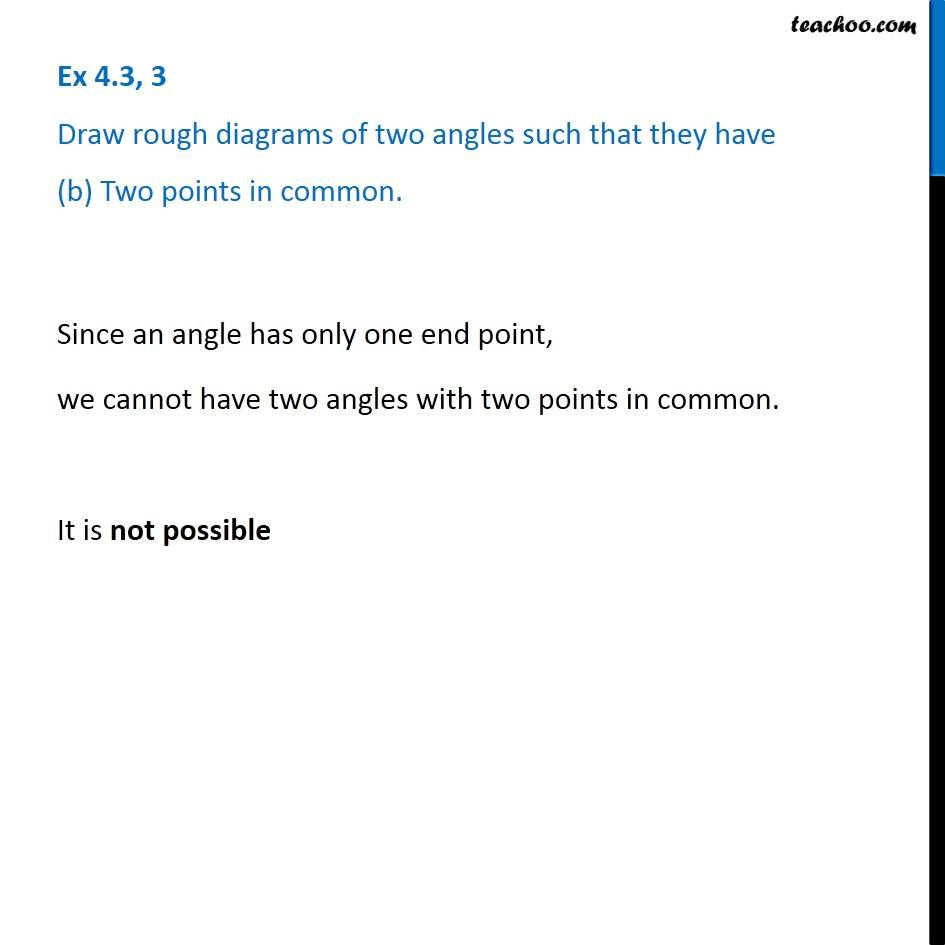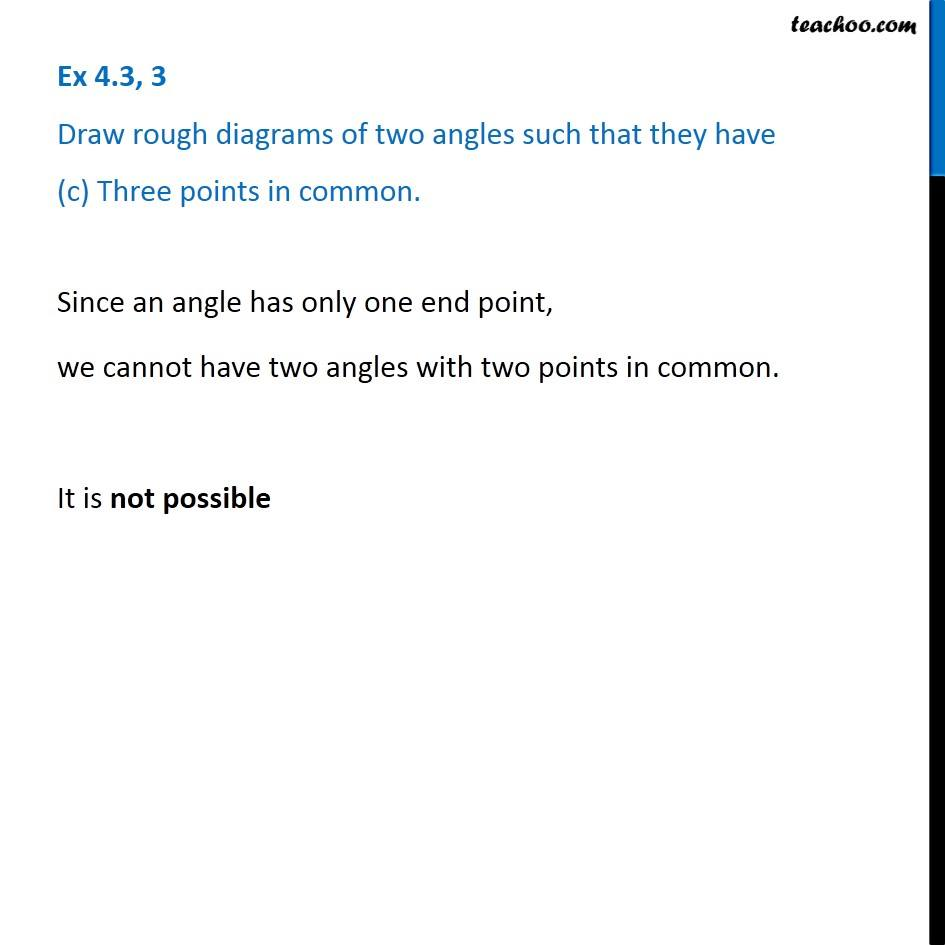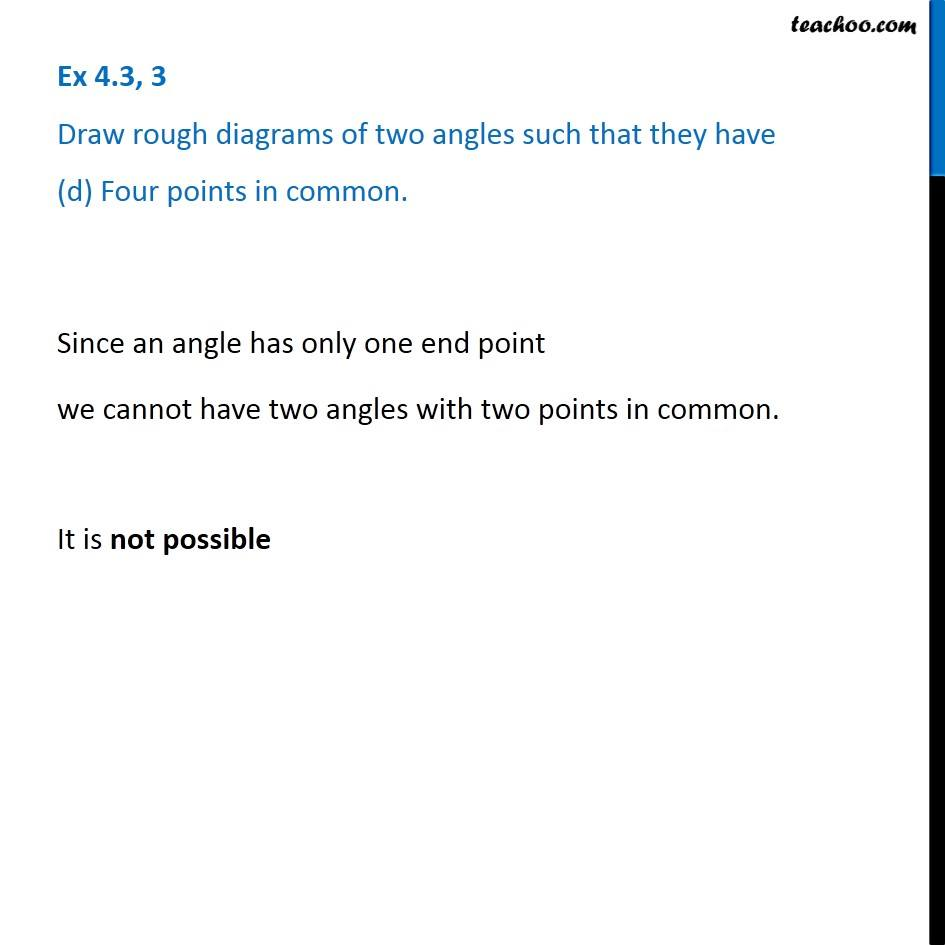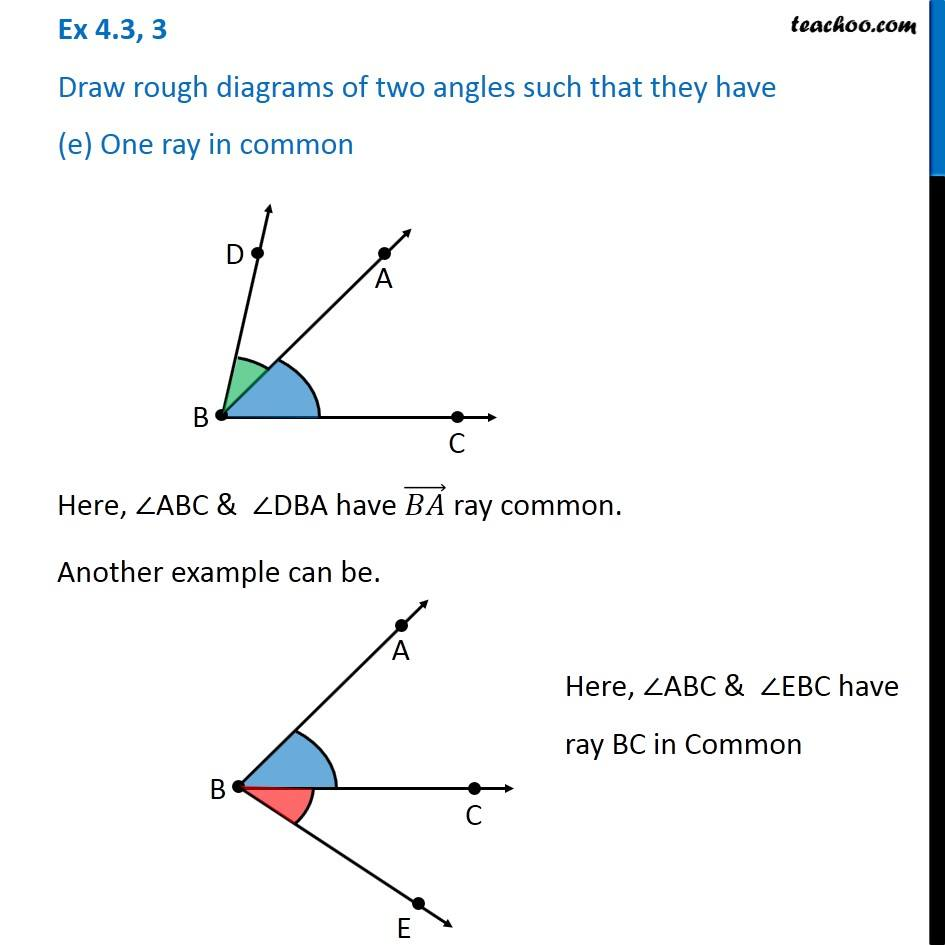Subscribe to our Youtube Channel - https://you.tube/teachoo

1. Chapter 4 Class 6 Basic Geometrical Ideas
2. Serial order wise
3. Ex 4.3

Transcript

Ex 4.3, 3 Draw rough diagrams of two angles such that they have (a) One point in common. If two angles have a common point, then their end point is the same Here, ABC Ex 4.3, 3 Draw rough diagrams of two angles such that they have (b) Two points in common. Since an angle has only one end point, we cannot have two angles with two points in common. It is not possible Ex 4.3, 3 Draw rough diagrams of two angles such that they have (c) Three points in common. Since an angle has only one end point, we cannot have two angles with two points in common. It is not possible Ex 4.3, 3 Draw rough diagrams of two angles such that they have (d) Four points in common. Since an angle has only one end point we cannot have two angles with two points in common. It is not possible Ex 4.3, 3 Draw rough diagrams of two angles such that they have (e) One ray in common Here, ABC & DBA have ( ) ray common. Another example can be. Here, ABC & EBC have ray BC in Common

Ex 4.3